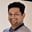Related Tags

java
communitycreator

# How to code Fibonacci sequence using the bottom-up approach of DPTarun Telang

## The idea behind the bottom-up approach of Dynamic Programming (DP)

1. Break the problem into smaller and simpler problems.

2. Solve the smaller and simpler problems obtained in the step above.

3. As we solve these smaller and simpler problems, we store the solutions obtained in the memory so that we can reuse them later while solving bigger and more complex problems.

4. Solve the bigger and more complex problems using the previously obtained solution from the memory.

## Bottom-up approach in action

Let’s see this approach in action using the example of the Fibonacci series.

### Problem

Given an integer n, print the first n integers of the Fibonacci series.

Before looking into my solution below, please try to solve this by yourself.

### Solution

Here we solve the problem for smaller numbers first and store them in an array. Later, we will use these values to calculate larger values from the Fibonacci series.

Below is the code:

import java.util.*;

class Main {

// array to store fibonacci series.
static int[] fib;

public static void fibonacci(int n) {
fib = new int[n+1];
fib = 1;
fib = 1;

for(int i = 2; i <= n; i++) {
// fib[i-2] and fib[i-1] were calculated
// in previous iterations.
fib[i] = fib[i-1] + fib[i-2];
}

// printing the fibonacci series
System.out.println(Arrays.toString(fib));
}

public static void main(String[] args) {
fibonacci(5);
}
}
Program to print Fibonacci series

## Analysis

### Time complexity

In the code above, we have a single for loop with (n - 1) iterations O(n - 1) and a call to Arrays.toString(fib) method that will have complexity of O(n). The overall time complexity of the program above is:

O(n - 1) + O(n) = O( 2 * n) = O(n)

### Space Complexity

Since we need a single array with n+1 elements to store the results, the total space complexity of the program is:

O(n + 1) = O (n)

RELATED TAGS

java
communitycreator

CONTRIBUTORTarun Telang
RELATED COURSES

View all Courses

Keep Exploring

Learn in-demand tech skills in half the time